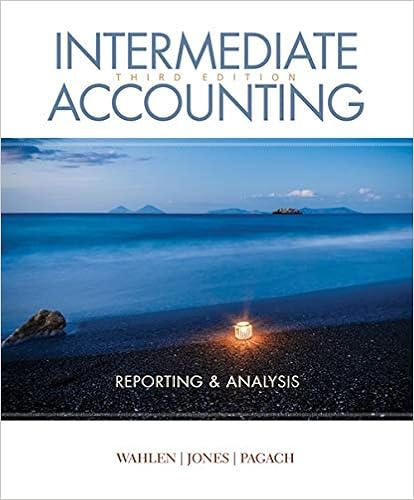# Standard Costing - PERFORMANCE EVALUATION THROUGH STANDARD...

• Notes
• 36
• 96% (45) 43 out of 45 people found this document helpful

This preview shows page 1 - 3 out of 36 pages.

##### We have textbook solutions for you!
The document you are viewing contains questions related to this textbook.The document you are viewing contains questions related to this textbook.
Chapter 7 / Exercise P7-5
Intermediate Accounting: Reporting and Analysis
Jones/WahlenExpert Verified
PERFORMANCE EVALUATION THROUGHSTANDARD COSTSTRUE-FALSE STATEMENTS1.Inventories cannot be valued at standard cost in financial statements.2.Standard cost is the industry average cost for a particular item.3.A standard is a unit amount, whereas a budget is a total amount.4.Standard costs may be incorporated into the accounts in the general ledger.5.An advantage of standard costs is that they simplify costing of inventories and reduce clerical costs.6.Setting standard costs is relatively simple because it is done entirely by accountants.7.Normal standards should be rigorous but attainable.8.Actual costs that vary from standard costs always indicate inefficiencies.9.Ideal standards will generally result in favorable variances for the company.10.Normal standards incorporate normal contingencies of production into the standards.11.Once set, normal standards should not be changed during the year.12.In developing a standard cost for direct materials, a price factor and a quantity factor must be considered.13.A direct labor price standard is frequently called the direct labor efficiency standard.14.The standard predetermined overhead rate must be based on direct labor hours as the standard activity index.15.Standard cost cards are the subsidiary ledger for the Work in Process account in a standard cost system.16.A variance is the difference between actual costs and standard costs.17.If actual costs are less than standard costs, the variance is favorable.18.A materials quantity variance is calculated as the difference between the standard direct materials price and the actual direct materials price multiplied by the actual quantity of direct materials used.19.An unfavorable labor quantity variance indicates that the actual number of direct labor hours worked was greater than the number of direct labor hours that should have been worked for the output attained.
##### We have textbook solutions for you!
The document you are viewing contains questions related to this textbook.The document you are viewing contains questions related to this textbook.
Chapter 7 / Exercise P7-5
Intermediate Accounting: Reporting and Analysis
Jones/WahlenExpert Verified
Test Bank for Managerial Accounting, Second Edition20.Standard cost + price variance + quantity variance = Budgeted cost.21.The overhead controllable variance relates primarily to fixed overhead costs.22.The overhead volume variance relates only to fixed overhead costs.23.If production exceeds normal capacity, the overhead volume variance will be favorable.24.There could be instances where the production department is responsible for a direct materials price variance.25.The starting point for determining the causes of an unfavorable materials price variance is the purchasing department.26.A two-variance analysis of overhead consists of a controllable variance and a volume variance.27.Variance analysis facilitates the principle of "management by exception."*28.A credit to a Materials Quantity Variance account indicates that the actual quantity of direct materials used was greater than the standard quantity of direct materials allowed.
•••